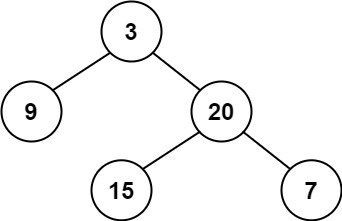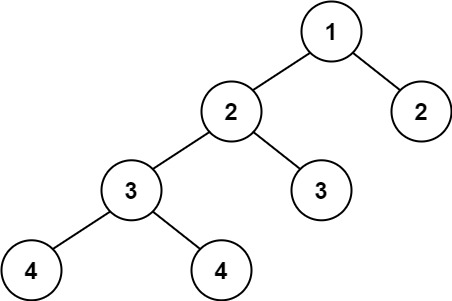# 110. Balanced Binary Tree

catepk·2021년 8월 22일
0

## LeetCode

목록 보기
48/88110. Balanced Binary Tree

### 문제

Given a binary tree, determine if it is height-balanced.

For this problem, a height-balanced binary tree is defined as:

a binary tree in which the left and right subtrees of every node differ in height by no more than 1.

Example 1:Input: root = [3,9,20,null,null,15,7]
Output: true

Example 2:Input: root = [1,2,2,3,3,null,null,4,4]
Output: false

Example 3:

Input: root = []
Output: true

Constraints:

The number of nodes in the tree is in the range [0, 5000].
-10^4 <= Node.val <= 10^4

### 풀이

# Definition for a binary tree node.
# class TreeNode:
#     def __init__(self, val=0, left=None, right=None):
#         self.val = val
#         self.left = left
#         self.right = right
class Solution:
def isBalanced(self, root: Optional[TreeNode]) -> bool:
def check(root):
if not root:
return 0

left = check(root.left)
right = check(root.right)
if left == -1 or right == -1 or abs(left - right) > 1:
return -1
return max(left, right) + 1
return check(root) != -1